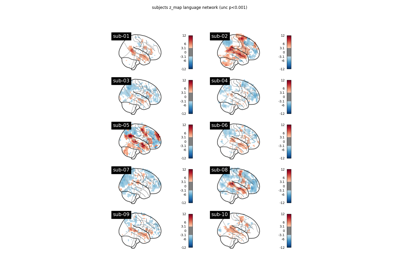Note

This page is a reference documentation. It only explains the class signature, and not how to use it. Please refer to the user guide for the big picture.

# nilearn.glm.second_level.SecondLevelModel#

class nilearn.glm.second_level.SecondLevelModel(mask_img=None, target_affine=None, target_shape=None, smoothing_fwhm=None, memory=Memory(location=None), memory_level=1, verbose=0, n_jobs=1, minimize_memory=True)[source]#

Implement the General Linear Model for multiple subject fMRI data.

Parameters:
mask_imgNiimg-like, `NiftiMasker` or `MultiNiftiMasker`, optional

Mask to be used on data. If an instance of masker is passed, then its mask will be used. If no mask is given, it will be computed automatically by a `MultiNiftiMasker` with default parameters. Automatic mask computation assumes first level imgs have already been masked.

target_affine`numpy.ndarray`, default=None

If specified, the image is resampled corresponding to this new affine. target_affine can be a 3x3 or a 4x4 matrix.

Note

This parameter is passed to `nilearn.image.resample_img`.

target_shape`tuple` or `list`, default=None

If specified, the image will be resized to match this new shape. len(target_shape) must be equal to 3.

Note

If target_shape is specified, a target_affine of shape (4, 4) must also be given.

Note

This parameter is passed to `nilearn.image.resample_img`.

smoothing_fwhm`float`, optional.

If smoothing_fwhm is not None, it gives the full-width at half maximum in millimeters of the spatial smoothing to apply to the signal.

memoryinstance of `joblib.Memory`, `str`, or `pathlib.Path`

Used to cache the masking process. By default, no caching is done. If a `str` is given, it is the path to the caching directory.

memory_level`int`, default=1

Rough estimator of the amount of memory used by caching. Higher value means more memory for caching. Zero means no caching.

verbose`int`, default=0

Verbosity level (0 means no message). If 0 prints nothing. If 1 prints final computation time. If 2 prints masker computation details.

n_jobs`int`, default=1

The number of CPUs to use to do the computation. -1 means ‘all CPUs’.

minimize_memory`bool`, optional

Gets rid of some variables on the model fit results that are not necessary for contrast computation and would only be useful for further inspection of model details. This has an important impact on memory consumption. Default=True.

__init__(mask_img=None, target_affine=None, target_shape=None, smoothing_fwhm=None, memory=Memory(location=None), memory_level=1, verbose=0, n_jobs=1, minimize_memory=True)[source]#
fit(second_level_input, confounds=None, design_matrix=None)[source]#

Fit the second-level GLM.

1. create design matrix

2. do a masker job: fMRI_data -> Y

3. fit regression to (Y, X)

Parameters:
second_level_input`list` of `FirstLevelModel` objects or `pandas.DataFrame` or `list` of Niimg-like objects.
• Giving `FirstLevelModel` objects will allow to easily compute the second level contrast of arbitrary first level contrasts thanks to the first_level_contrast argument of `compute_contrast`. Effect size images will be computed for each model to contrast at the second level.

• If a `DataFrame`, then it has to contain subject_label, map_name and effects_map_path. It can contain multiple maps that would be selected during contrast estimation with the argument first_level_contrast of `compute_contrast`. The `DataFrame` will be sorted based on the subject_label column to avoid order inconsistencies when extracting the maps. So the rows of the automatically computed design matrix, if not provided, will correspond to the sorted subject_label column.

• If a `list` of Niimg-like objects then this is taken literally as Y for the model fit and design_matrix must be provided.

confounds`pandas.DataFrame`, optional

Must contain a `subject_label` column. All other columns are considered as confounds and included in the model. If `design_matrix` is provided then this argument is ignored. The resulting second level design matrix uses the same column names as in the given `DataFrame` for confounds. At least two columns are expected, `subject_label` and at least one confound.

design_matrix`pandas.DataFrame`, optional

Design matrix to fit the GLM. The number of rows in the design matrix must agree with the number of maps derived from `second_level_input`. Ensure that the order of maps given by a `second_level_input` list of Niimgs matches the order of the rows in the design matrix.

compute_contrast(second_level_contrast=None, first_level_contrast=None, second_level_stat_type=None, output_type='z_score')[source]#

Generate different outputs corresponding to the contrasts provided e.g. z_map, t_map, effects and variance.

Parameters:
second_level_contrast`str` or `numpy.ndarray` of shape(n_col), optional

Where n_col is the number of columns of the design matrix. The string can be a formula compatible with `pandas.DataFrame.eval`. Basically one can use the name of the conditions as they appear in the design matrix of the fitted model combined with operators +- and combined with numbers with operators +-*/. The default None is accepted if the design matrix has a single column, in which case the only possible contrast array((1)) is applied; when the design matrix has multiple columns, an error is raised.

first_level_contrast`str` or `numpy.ndarray` of shape (n_col) with respect to `FirstLevelModel`, optional
• In case a `list` of `FirstLevelModel` was provided as `second_level_input`, we have to provide a contrast to apply to the first level models to get the corresponding list of images desired, that would be tested at the second level.

• In case a `DataFrame` was provided as `second_level_input` this is the map name to extract from the `DataFrame` `map_name` column. It has to be a ‘t’ contrast.

second_level_stat_type{‘t’, ‘F’} or None, optional

Type of the second level contrast. Default=None.

output_type{‘z_score’, ‘stat’, ‘p_value’, ‘effect_size’, ‘effect_variance’, ‘all’}, optional

Type of the output map. Default=’z-score’.

Returns:
output_image`Nifti1Image`

The desired output image(s). If `output_type == 'all'`, then the output is a dictionary of images, keyed by the type of image.

fit_transform(X, y=None, **fit_params)#

Fit to data, then transform it.

Fits transformer to X and y with optional parameters fit_params and returns a transformed version of X.

Parameters:
Xarray-like of shape (n_samples, n_features)

Input samples.

yarray-like of shape (n_samples,) or (n_samples, n_outputs), default=None

Target values (None for unsupervised transformations).

**fit_paramsdict

Returns:
X_newndarray array of shape (n_samples, n_features_new)

Transformed array.

generate_report(contrasts, title=None, bg_img='MNI152TEMPLATE', threshold=3.09, alpha=0.001, cluster_threshold=0, height_control='fpr', min_distance=8.0, plot_type='slice', display_mode=None, report_dims=(1600, 800))[source]#

Return a `HTMLReport` which shows all important aspects of a fitted GLM.

The `HTMLReport` can be opened in a browser, displayed in a notebook, or saved to disk as a standalone HTML file.

The GLM must be fitted and have the computed design matrix(ces).

Note

The `FirstLevelModel` or `SecondLevelModel` must have been fitted prior to calling `generate_report`.

Parameters:
contrasts

Contrasts information for a `FirstLevelModel` or `SecondLevelModel`.

Example:

Dict of contrast names and coefficients, or list of contrast names or list of contrast coefficients or contrast name or contrast coefficient

Each contrast name must be a string. Each contrast coefficient must be a list or numpy array of ints.

Contrasts are passed to `contrast_def` for `FirstLevelModel` through `compute_contrast`, and `second_level_contrast` for `SecondLevelModel` through `compute_contrast`.

title`str`, optional
• If a `str`, it represents the web page’s title and primary heading, model type is sub-heading.

• If `None`, page titles and headings are autogenerated using contrast names.

bg_imgNiimg-like object, optional

Default is the MNI152 template (Default=’MNI152TEMPLATE’) See Input and output: neuroimaging data representation. The background image for mask and stat maps to be plotted on upon. To turn off background image, just pass “bg_img=None”.

threshold`float`, optional

Cluster forming threshold in same scale as `stat_img` (either a t-scale or z-scale value). Used only if `height_control` is `None`. Default=3.09

alpha`float`, optional

Number controlling the thresholding (either a p-value or q-value). Its actual meaning depends on the `height_control` parameter. This function translates alpha to a z-scale threshold. Default=0.001

cluster_threshold`int`, optional

Cluster size threshold, in voxels. Default=0

height_control`str` or None, optional

False positive control meaning of cluster forming threshold: ‘fpr’, ‘fdr’, ‘bonferroni’ or `None`. Default=’fpr’.

min_distance`float`, optional

For display purposes only. Minimum distance between subpeaks in mm. Default=8mm.

plot_type{‘slice’, ‘glass’}, optional

Specifies the type of plot to be drawn for the statistical maps. Default=’slice’.

display_mode{‘ortho’, ‘x’, ‘y’, ‘z’, ‘xz’, ‘yx’, ‘yz’, ‘l’, ‘r’, ‘lr’, ‘lzr’, ‘lyr’, ‘lzry’, ‘lyrz’}, optional

Choose the direction of the cuts:

• ‘x’ - sagittal

• ‘y’ - coronal

• ‘z’ - axial

• ‘l’ - sagittal left hemisphere only

• ‘r’ - sagittal right hemisphere only

• ‘ortho’ - three cuts are performed in orthogonal directions

Default is ‘z’ if `plot_type` is ‘slice’; ‘ortho’ if `plot_type` is ‘glass’.

report_dimsSequence[ `int`, `int` ], optional

Specifies width, height (in pixels) of report window within a notebook. Only applicable when inserting the report into a Jupyter notebook. Can be set after report creation using `report.width`, `report.height`. Default=(1600, 800).

Returns:
report_text`HTMLReport`

Contains the HTML code for the GLM report.

Get metadata routing of this object.

Please check User Guide on how the routing mechanism works.

Returns:

A `MetadataRequest` encapsulating routing information.

get_params(deep=True)#

Get parameters for this estimator.

Parameters:
deepbool, default=True

If True, will return the parameters for this estimator and contained subobjects that are estimators.

Returns:
paramsdict

Parameter names mapped to their values.

predicted()[source]#

Transform voxelwise predicted values to the same shape as the input Nifti1Image(s).

Returns:
outputlist

A list of Nifti1Image(s).

r_square()[source]#

Transform voxelwise r-squared values to the same shape as the input Nifti1Image(s).

Returns:
outputlist

A list of Nifti1Image(s).

residuals()[source]#

Transform voxelwise residuals to the same shape as the input Nifti1Image(s).

Returns:
outputlist

A list of Nifti1Image(s).

set_fit_request(*, confounds='\$UNCHANGED\$', design_matrix='\$UNCHANGED\$', second_level_input='\$UNCHANGED\$')#

Request metadata passed to the `fit` method.

Note that this method is only relevant if `enable_metadata_routing=True` (see `sklearn.set_config`). Please see User Guide on how the routing mechanism works.

The options for each parameter are:

• `True`: metadata is requested, and passed to `fit` if provided. The request is ignored if metadata is not provided.

• `False`: metadata is not requested and the meta-estimator will not pass it to `fit`.

• `None`: metadata is not requested, and the meta-estimator will raise an error if the user provides it.

• `str`: metadata should be passed to the meta-estimator with this given alias instead of the original name.

The default (`sklearn.utils.metadata_routing.UNCHANGED`) retains the existing request. This allows you to change the request for some parameters and not others.

New in version 1.3.

Note

This method is only relevant if this estimator is used as a sub-estimator of a meta-estimator, e.g. used inside a `Pipeline`. Otherwise it has no effect.

Parameters:
confoundsstr, True, False, or None, default=sklearn.utils.metadata_routing.UNCHANGED

Metadata routing for `confounds` parameter in `fit`.

design_matrixstr, True, False, or None, default=sklearn.utils.metadata_routing.UNCHANGED

Metadata routing for `design_matrix` parameter in `fit`.

second_level_inputstr, True, False, or None, default=sklearn.utils.metadata_routing.UNCHANGED

Metadata routing for `second_level_input` parameter in `fit`.

Returns:
selfobject

The updated object.

set_output(*, transform=None)#

Set output container.

See Introducing the set_output API for an example on how to use the API.

Parameters:
transform{“default”, “pandas”}, default=None

Configure output of transform and fit_transform.

• “default”: Default output format of a transformer

• “pandas”: DataFrame output

• None: Transform configuration is unchanged

Returns:
selfestimator instance

Estimator instance.

set_params(**params)#

Set the parameters of this estimator.

The method works on simple estimators as well as on nested objects (such as `Pipeline`). The latter have parameters of the form `<component>__<parameter>` so that it’s possible to update each component of a nested object.

Parameters:
**paramsdict

Estimator parameters.

Returns:
selfestimator instance

Estimator instance.

## Examples using `nilearn.glm.second_level.SecondLevelModel`#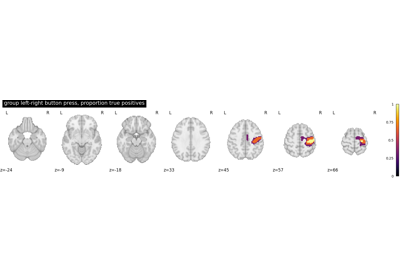Second-level fMRI model: true positive proportion in clusters

Second-level fMRI model: true positive proportion in clusters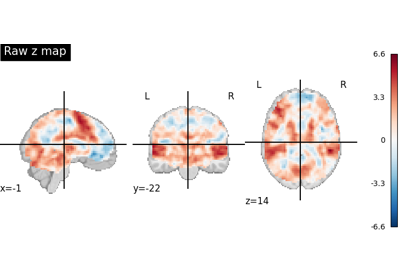Statistical testing of a second-level analysis

Statistical testing of a second-level analysis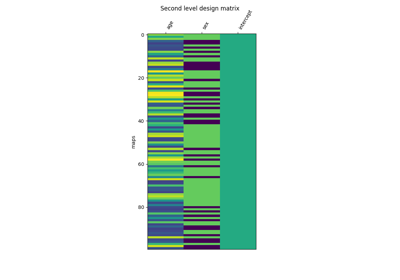Voxel-Based Morphometry on OASIS dataset

Voxel-Based Morphometry on OASIS dataset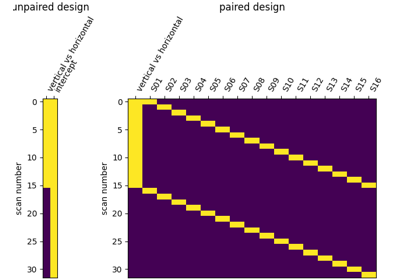Second-level fMRI model: two-sample test, unpaired and paired

Second-level fMRI model: two-sample test, unpaired and paired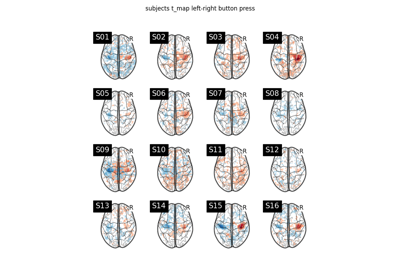Second-level fMRI model: one sample test

Second-level fMRI model: one sample test